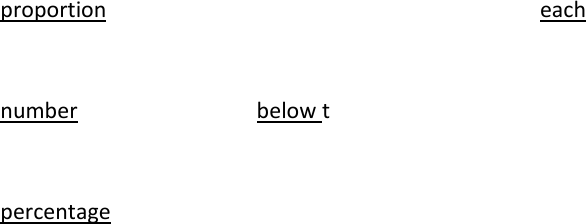# PSY201H1 Chapter Notes - Chapter 3: Percentile Rank, Cumulative Frequency Analysis, Frequency Distribution

11 views3 pages
Page:
of 3Understanding Statistics Chapter 3
PSY201
Grouping Scores
Frequency distribution of grouped scores
How scores are to be presented when there are many so that we can see the shape
of the distribution
To construct a frequency distribution of grouped scores
Find the range of scores
Highest sore minus the lowest score
Determine the width of each class interval (i)
i= range/# of class intervals
Remember to count EACH unit, so 50-55 isn’t 5 units wide, it’s 6 units wide
List the limits of each class interval, placing the interval containing the lowest
score value at the bottom
Start with the lowest, which must contain the lower limit of the interval
Make the lower limit divisible by i! So if the lowest unit is 42, start with 40 if
the i is 5 or 10
Tally the raw scores into the appropriate class intervals
Add the tallies of each interval to obtain the interval frequency
Relative Frequency, Cumulative Frequency, and Cumulative Percentage Distributions
Relative frequency distribution
Indicates the proportion of the total number of scores that occurs in each interval
Relative frequency = f/N [frequency divided by total number of scores]
Cumulative frequency distribution
Indicates the number of scores that fall below the upper limit of each interval
Add the frequency of that interval to the frequencies of all the intervals below it
Cumulative percentage distribution
Indicates the percentage of scores that fall below the upper real limit of each
interval
Convert cumulative frequencies to cumulative percentage
cum%=[(cumf)/N] X100
Percentiles
A percentile, or percentile point, is the value on the measures scale below which a
specified percentage of scores in the distribution fall
A measurement of relative standing, and to compare an individual score to that of a
reference group
Notation: for 50th percentile point, we write P50
Means: Value below which 50% of the other values fall
To find a percentile point
Determine the frequency of the scores below the percentile point = cumfp
cumfp= (% of scores below this percentile point) XN
For example, if there are 70 scores:
cumfp = (.50) X 70 = 35
Determine the lower real limit of the interval containing the percentile point
XL = real lower limit
Can be done by comparing the cumfp with the cumulative frequency for each
interval
Determine the number of additional scores we must acquire in the interval to reach
the percentile point
Number of additional scores = cumfp-cumfL
cumfL= frequency of scores below the lower real limit of the interval
containing the percentile point
Determine the number of additional units into the interval we must go to acquire
the additional number of units
Additional units = (number of units per score) X Number of additional units
(i/fi) X number of additional units
fi is the number of scores in the interval
i/fi is the number of units per score for the interval
Percentile Rank
Percentile rank is a score is the percentage of scores with values lower than the score in
question
Equation = {[cumfL + (fi/i)(X-XL)]N} X100
Graphing Frequency Distribution
Bar Graph
Best for nominal or ordinal data
Similar to a histogram, but there is a space between each bar that shows the
separation of the categories
Histogram
Best for interval or ratio data
Similar to a bar graph, but there is no space between each bar, just a line that shows
the real limit of the unit
Frequency Polygon
Best for interval or ration data
Instead of bars, there are points at the intervals and a line that connects each of the
dots
Cumulative Percentage Curve
Symmetrical
When folded in half, the two sides collide
If a curve is not symmetrical, it is skewed
Skewed
Positively skewed
When most of the scores occur at the lower values of the horizontal axis and
the curve tails off towards the higher end
Negatively skewed
When most of the scores occur at the higher values of the horizontal axis and
the curve tails off towards the lower end